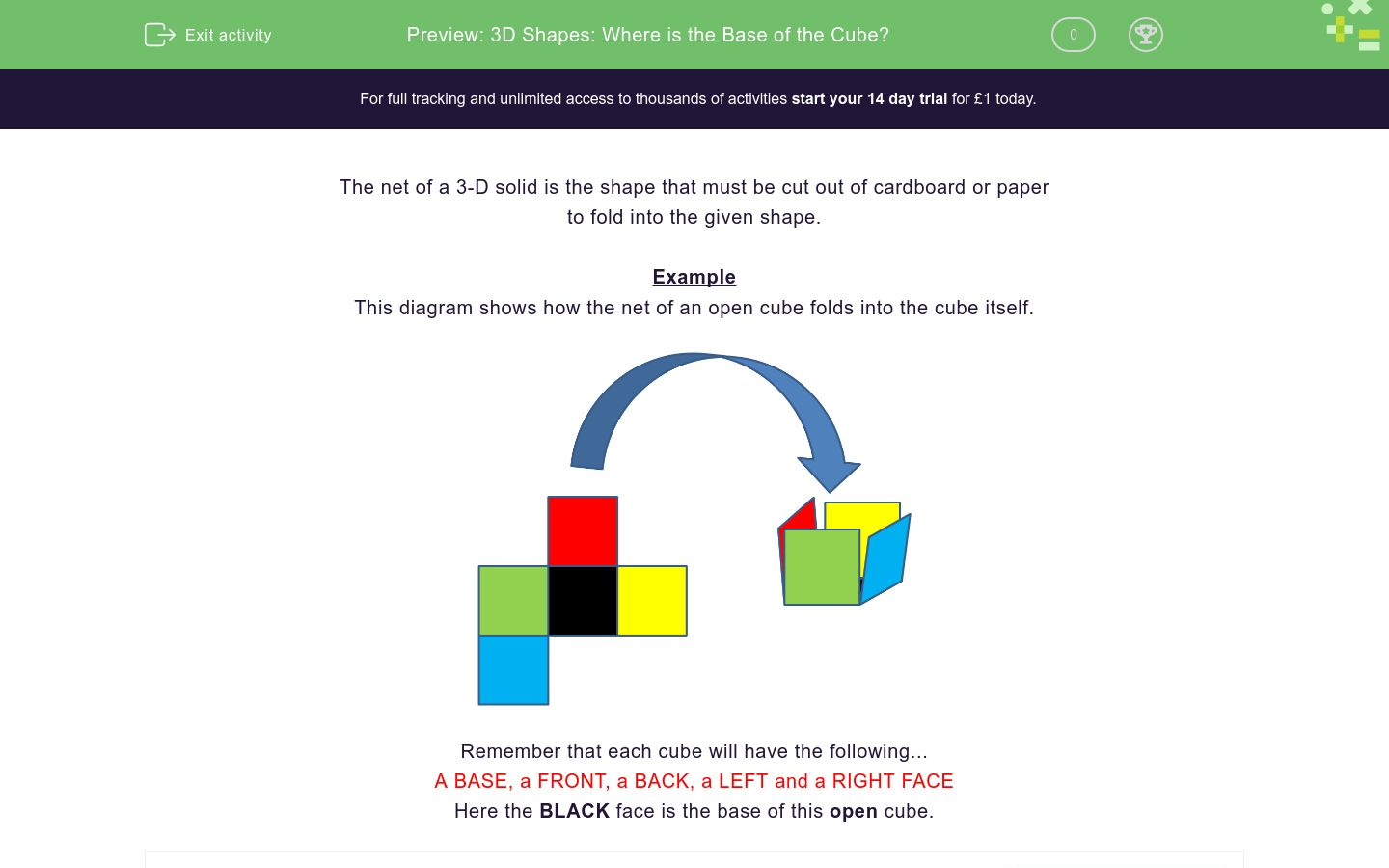# 3D Shapes: Where is the Base of the Cube?

In this worksheet, students identify the bases of open cubes from their nets.Key stage:  KS 2

Curriculum topic:   Geometry: Properties of Shapes

Curriculum subtopic:   Identify 3D Shapes

Difficulty level:### QUESTION 1 of 10

The net of a 3-D solid is the shape that must be cut out of cardboard or paper

to fold into the given shape.

Example

This diagram shows how the net of an open cube folds into the cube itself.Remember that each cube will have the following...

A BASE, a FRONT, a BACK, a LEFT and a RIGHT FACE

Here the BLACK face is the base of this open cube.

---- OR ----